# Quick Maths Lesson Starters

If you are looking for a very quick lesson starter because you want to get on with the main part of the lesson here are some ideas.

They have been selected because they do the job of getting the students focussed but they mostly are direct questions with a given answer to provide closure. If you choose to use the optional student activity the time required will need to be extended.

Main Page

### Quick Starters: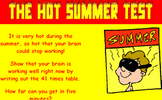Hot Summer Test: Write out a large times table. Get as far as possible in 5 minutes. An optional student activity is linked from the bottom of this starter page called Times Tables. A collection of activities to help you learn your times tables in only 5 days..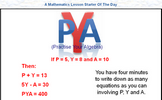PYA: You have four minutes to write down as many equations as you can involving the given letters. An optional student activity is linked from the bottom of this starter page called Substitution. Substitute the given values into the algebraic expressions..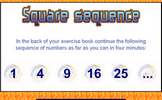Square Sequence: Write out as many square numbers as possible in 4 minutes. An optional student activity is linked from the bottom of this starter page called Don't Shoot The Square. You will need to be quick on the draw to shoot all of the numbers except the square numbers..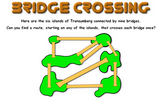Bridge Crossing: The classic puzzle of finding a route which crosses each bridge once. An optional student activity is linked from the bottom of this starter page called Window View. Drag the 20 flowers into the gardens so that 9 flowers are visible from each window of the house..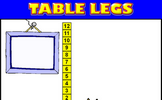Table Legs: Learn an unusual times table from the strategic finger moving up and down the 'Table Leg'! An optional student activity is linked from the bottom of this starter page called Times Tables. A collection of activities to help you learn your times tables in only 5 days..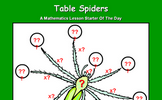Table Spiders: Multiply the number on the spider's back by the numbers next to its legs. An optional student activity is linked from the bottom of this starter page called Times Tables. A collection of activities to help you learn your times tables in only 5 days..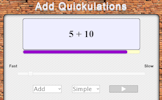Add Quickulations: Calculations appear on the screen every few seconds. This mental arithmetic starter provides pace to the start of the Maths lesson. An optional student activity is linked from the bottom of this starter page called Basic Addition. A self-marking exercise on addition with increasing levels of difficulty..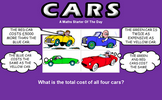Cars: Calculate the total cost of four cars from the information given. An optional student activity is linked from the bottom of this starter page called Mathopoly. A game of buying and selling property with maths questions thrown in for good measure..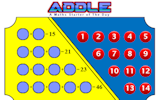Addle: Arrange the numbers 1 to 14 in the circles so that the sums are correct. An optional student activity is linked from the bottom of this starter page called Addle. Arrange the numbers from 1 to 14 in the spaces to make the sums correct. How fast can you do it?.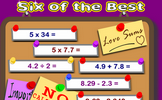Six of the Best: Six calculations to perform without a calculator. An optional student activity is linked from the bottom of this starter page called Number Skills Inventory. A checklist of basic numeracy techniques that every pupil should know..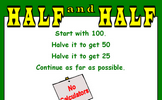Half and Half: Start with 100. Halve it to get 50. Halve that to get 25. Continue as far as possible. An optional student activity is linked from the bottom of this starter page called Basic Division. A self-marking exercise on dividing numbers of up to four digits by one or two-digit whole numbers using the formal written methods of short and long division.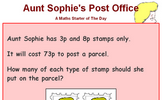Aunt Sophie's Post Office: Work out the number of stamps needed to post a parcel. An optional student activity is linked from the bottom of this starter page called Stamp Sticking. Drag stamps onto the envelopes to make the exact postage as shown at the top left of each envelope..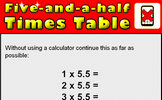5.5 Times Table: Write out the 5.5 times table as far as possible. An optional student activity is linked from the bottom of this starter page called Flash Tables. A never ending sequence of times tables questions to be projected on to a whiteboard or screen..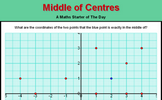Middle of Centres: The blue point is exactly in the middle of two red points. What are their coordinates? An optional student activity is linked from the bottom of this starter page called Coordinates. A self marking exercise on identifying coordinates culminating in finding the mid point of two given points..Guitar For Sale: A question about the profit made when buying and selling a guitar. An optional student activity is linked from the bottom of this starter page called Aunt Lucy's Legacy. Decide which of the four schemes Aunt Lucy proposes will provide the most money. This investigation involves the sum of sequences as well as considering life expectancy..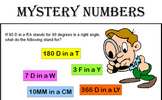Mystery Numbers: Can you recognise the mystery numbers from the clues? An optional student activity is linked from the bottom of this starter page called Mystery Numbers. If '7 D in a W' stands for 7 days in a week, what do you think these mystery numbers are?.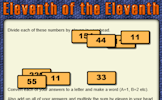Eleventh of the Eleventh: Practise multiplying and dividing by eleven in your head. An optional student activity is linked from the bottom of this starter page called Eleven In Your Head. Multiply numbers by eleven in your head using the tips and tricks provided..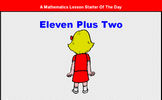Eleven Plus Two: Rearrange the letters in the expression 'Eleven Plus Two' to make another expression with the same value. An optional student activity is linked from the bottom of this starter page called Mixpressions. Arrange the cards to create a valid mathematical statement..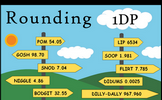Rounding 1dp: Round off the given numbers to 1 decimal place then add the answers together. An optional student activity is linked from the bottom of this starter page called Rounding DP. A self marking exercise requiring students to round numbers to a given number of decimal places..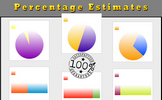Estimating Percentages: Estimate the percentages of full circles and rectangles the sectors represent. An optional student activity is linked from the bottom of this starter page called Estimating Percentages. Estimate the percentages represented by the diagrams..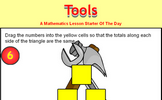Tool Triangle: Place the numbers on the triangle so that the totals along each of the sides are equal. An optional student activity is linked from the bottom of this starter page called Tools. In how many different ways can the numbers be arranged to give the same totals?.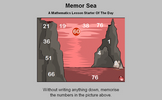Memor Sea: Twelve numbers in a seascape need to be memorised in order to answer the five questions. An optional student activity is linked from the bottom of this starter page called Screen Test. Memorise the mathematical facts in the video then answer the ten quiz questions..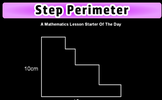Perimeter: Is it possible to work out the perimeter of this shape if not all the side lengths are given? An optional student activity is linked from the bottom of this starter page called Online Logo. An online version of the Logo programming language with 30 mathematical challenges..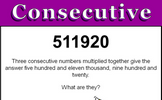Consecutive: Three consecutive numbers multiplied together give a given product. Students are asked to figure out what the numbers are. An optional student activity is linked from the bottom of this starter page called Consecutive Numbers. Find the consective numbers that are added or multiplied to give the given totals.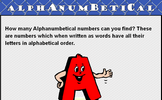Alphanumbetical: A challenge to find the number which when written as a word has all the letters in alphabetical order. An optional student activity is linked from the bottom of this starter page called Mathanagrams. The letters of mathematical words have been mixed up. Can you recognise them?.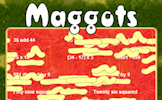Maggots: Do as many of the calculations as possible before the maggots infest! An optional student activity is linked from the bottom of this starter page called Maze. Each visitor to this page has a unique maze constructed for them to find their way through answering mathematical questions on the way..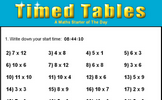Timed Tables: How fast can you answer 24 mixed times tables questions? An optional student activity is linked from the bottom of this starter page called Times Tables. A collection of activities to help you learn your times tables in only 5 days..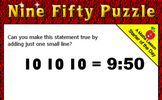9:50 Puzzle: A little lateral thinking might help you solve this puzzle. An optional student activity is linked from the bottom of this starter page called Stick Squares. Arrange the matches to form four squares instead of five..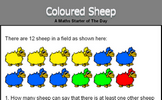Coloured Sheep: What is the probability of picking a red sheep from the sheep in the field? An optional student activity is linked from the bottom of this starter page called Likelihood. Arrange some statements in order according to the probability of them happening. Compare your opinion with thousands of others..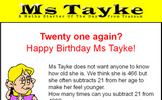Ms Tayke: How many times can you take one number from another? An optional student activity is linked from the bottom of this starter page called Ten Subtractions. Ten subtractions for you to do without a calculator..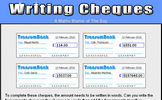Writing Cheques: Complete some imaginary cheques, the amount needs to be written in words. An optional student activity is linked from the bottom of this starter page called Numbers in Words. Find the five lettered mathematical words by matching numbers with their equivalent in words..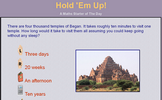Hold 'Em Up!: Everyone in the class holds up an item to show which is the correct answer to the multiple choice question. An optional student activity is linked from the bottom of this starter page called Pentransum. Mathematical questions with five possible answers. If you get 20 correct you can add your own question to the database..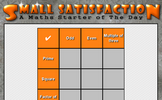Small Satisfaction: Arrange the digits one to nine in the grid so that they obey the row and column headings. An optional student activity is linked from the bottom of this starter page called Satisfy. Place the nine numbers in the table so they obey the row and column headings about the properties of the numbers..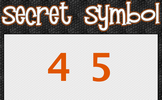Secret Symbol: Place a symbol between the four and the five to get a number greater than four and less than five. An optional student activity is linked from the bottom of this starter page called Thrice. Can you arrange all of the counters on the grid to form 10 lines of three counters?.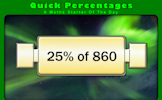Quick Percentages: Simple percentage questions appear on screen then fade every 8 seconds. This Starter is customisable. An optional student activity is linked from the bottom of this starter page called Express as a Percentage. This self-marking quiz requires you to work out what one quantity is as a percentage of a second quantity..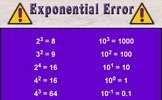Exponential Error: This lesson starter presents a number of statements about indices and pupils are asked if they can spot the mistake. An optional student activity is linked from the bottom of this starter page called Indices Pairs. The traditional pairs or pelmanism game adapted to test knowledge of indices..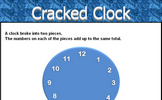Cracked Clock: How did the clock break if the numbers on each of the pieces added up to the same total? An optional student activity is linked from the bottom of this starter page called Cracked Clock Quiz. A self marking set of ten mathematical questions about a clock which cracked!.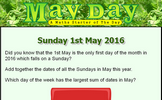May Day: Add together the dates of all the Thursdays in May this year. Which day sum is largest? An optional student activity is linked from the bottom of this starter page called Calendar Maths Investigation. Investigate the connection between the numbers in a T shape drawn on this month's calendar..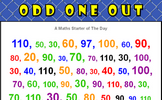Odd One Out: From the numbers given, find the one that is the odd one out. An optional student activity is linked from the bottom of this starter page called No Partner. Find which numbers in a given list do not combine with other numbers on the list to make a given sum..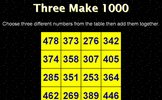Three Make 1000: Find three numbers from those given that add up to 1000. How many different sets of three numbers can be found? An optional student activity is linked from the bottom of this starter page called . .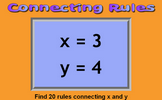Connecting Rules: Give 20 rules connecting x and y given their values. An optional student activity is linked from the bottom of this starter page called . .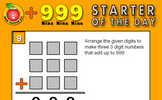Nine Nine Nine: Arrange the numbers 1-9 to make three 3 digit numbers that add up to 999. An optional student activity is linked from the bottom of this starter page called Nine Nine Nine. Use the digits 1 to 9 to make three 3 digit numbers which add up to 999..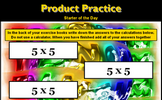Product Practice: Work out the answers to the given calculations then add the answers together. There are three different levels of difficulty. An optional student activity is linked from the bottom of this starter page called Ten Multiplications. Twelve multiplications for you to do without a calculator..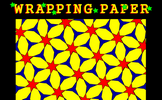Wrapping Paper: Find the order of rotational symmetry of the repeating pattern. An optional student activity is linked from the bottom of this starter page called Rotational Symmetry Pairs. The traditional pairs or pelmanism game adapted to test knowledge of Rotational Symmetry..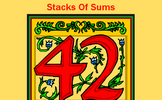Stacks of Sums: Write down many different types of calculations which give a particular answer. An optional student activity is linked from the bottom of this starter page called Make an Expression. Use the digits given to form an expression equalling the given total..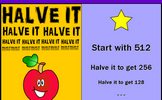Halve it: Start with 512. Halve it to get 256. Halve it to get 128. Continue as far as possible. An optional student activity is linked from the bottom of this starter page called Double Take. Merge two tiles into one tile to make a double. What is the largest double you can make?.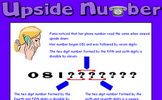Upside Number: Work out the phone number from the clues given. An optional student activity is linked from the bottom of this starter page called Square and Even. Arrange the numbers on the cards so that each of the three digit numbers formed horizontally are square numbers and each of the three digit numbers formed vertically are even..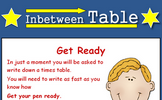Inbetween Table: Write down as many multiples of 3.5 as possible in 3.5 minutes. An optional student activity is linked from the bottom of this starter page called TablesMaster. How fast can you answer times table questions? This activity provides feedback to help you improve..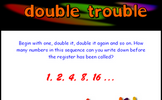Double Trouble: Begin with one, double it, double it again and so on. How many numbers in this sequence can you write down before the register has been called? An optional student activity is linked from the bottom of this starter page called Doubling Quiz. Drag the number cards onto their doubles. No calculators or writing allowed..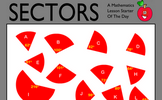Sectors: Work out which sectors fit together to make complete circles. Knowledge of the sum of the angles at a point will help find more than one correct solution to this puzzle. An optional student activity is linked from the bottom of this starter page called Angle Points. Apply the properties of angles at a point, angles on a straight line and vertically opposite angles..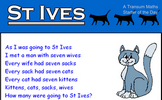St Ives: The traditional St Ives riddle. An optional student activity is linked from the bottom of this starter page called Maths Crossword. An interactive mathematical crossword for you to do online. Find the missing words from the given clues..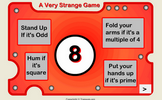A Very Strange Game: Four different actions depending on the number which appears. An optional student activity is linked from the bottom of this starter page called Satisfaction. This is quite a challenging number grouping puzzle requiring a knowledge of prime, square and triangular numbers..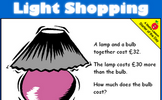Light Shopping: A lamp and a bulb together cost 32 pounds. The lamp costs 30 pounds more than the bulb. How much does the bulb cost? An optional student activity is linked from the bottom of this starter page called Algebra In Action. Real life problems adapted from an old Mathematics textbook which can be solved using algebra..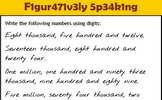Figuratively Speaking: Write the numbers written as words using digits. An optional student activity is linked from the bottom of this starter page called Words in Digits. Write the numbers given in words as digits and vice versa..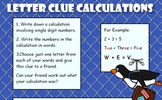Letter Clue Calculations: Work out what the calculations might be from the letter clues. An optional student activity is linked from the bottom of this starter page called Code Cracker. Crack the code by finding out which letters replace the encrypted letters in the text given. There are lots of hints provided about code breaking techniques..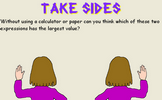Take Sides: Put up your right hand or left hand depending on the expressions that appears. An optional student activity is linked from the bottom of this starter page called Digivide. Arrange the numbers from 1 to 6 in the spaces to make the calculation correct..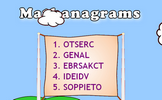Anagrams: Can you un-jumble these mathematical words? An optional student activity is linked from the bottom of this starter page called . .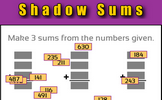Shadow Sums: Make sums from the three digit numbers given. An optional student activity is linked from the bottom of this starter page called Twelve Sums. A self-marking exercise on addition with increasing levels of difficulty..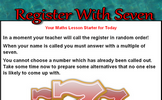Register: When the register is called answer with a multiple of 7. An optional student activity is linked from the bottom of this starter page called . .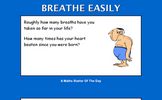Breathe Easily: How many breaths have you taken in your lifetime? An optional student activity is linked from the bottom of this starter page called Rounding SF. A self marking exercise requiring students to round numbers to a given number of significant figures..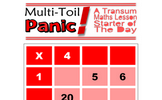Multiplication Grid: Copy and complete the multiplication grid. The higher levels include negative numbers. An optional student activity is linked from the bottom of this starter page called Clouds. Can you work out which numbers are hidden behind the clouds in these calculations?.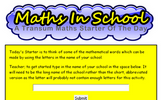Maths In School: Find mathematical words which can be made by using the letters in the name of your school? An optional student activity is linked from the bottom of this starter page called Mathanagrams. The letters of mathematical words have been mixed up. Can you recognise them?.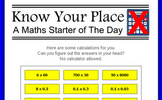Know Your Place: Without a calculator perform some calculations requiring a knowledge of place value. An optional student activity is linked from the bottom of this starter page called Know Your Place. Without a calculator perform some calculations requiring a knowledge of place value..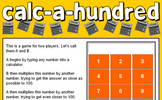Calc-A-Hundred: A game for two players requiring a calculator and thinking skills. An optional student activity is linked from the bottom of this starter page called Broken Calculator. Some of the buttons are missing from this calculator. Can you make the totals from 1 to 20?.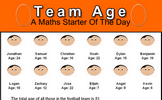Team Age: Work out who is in which team from the information given. An optional student activity is linked from the bottom of this starter page called Addle. Arrange the numbers from 1 to 14 in the spaces to make the sums correct. How fast can you do it?.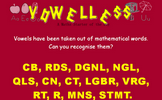Vowelless: Vowels have been taken out of mathematical words. Can you recognise them? An optional student activity is linked from the bottom of this starter page called Vowelless. Vowels have been taken out of mathematical words. Can you recognise them?.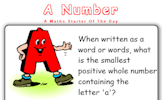A Number: When written as a word or words what is the smallest positive whole number containing the letter 'a'? An optional student activity is linked from the bottom of this starter page called Four Ever. Generate a number sequence based on the number of letters needed to spell the previous number..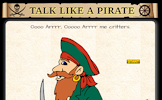Numbers in words: Write out in words some numbers writen as digits. An optional student activity is linked from the bottom of this starter page called Words in Digits. Write the numbers given in words as digits and vice versa..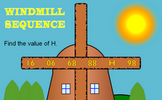Windmill Sequence: Find the value of the missing term of the sequence. It is easier than you may think! An optional student activity is linked from the bottom of this starter page called Scallywags and Scoundrels. Arrange the scallywags and scoundrels on the chairs so that the numbers of any two sitting next to each other add up to a prime number..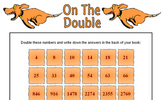On The Double: Double the numbers given in the table. An optional student activity is linked from the bottom of this starter page called Doubling Quiz. Drag the number cards onto their doubles. No calculators or writing allowed..

### Other

Is there anything you would have a regular use for that we don't feature here? Please let us know.

Contact Transum

#### Circle Equations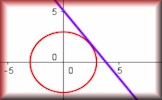Recognise and use the equation of a circle with centre at the origin and the equation of a tangent to a circle. So far this activity has been accessed 130 times and it is ready for you to enjoy!

### Feedback

Do you have any comments? It is always useful to receive feedback and helps make this free resource even more useful for those learning Mathematics anywhere in the world. Click here to enter your comments.For All:

©1997-2019 WWW.TRANSUM.ORG